### TRANSFORM Statement

TRANSFORM transform(variables </ t-options>)<transform(variables </ t-options>)…> ;

The TRANSFORM statement lists the variables to be analyzed (variables) and specifies the transformation (transform) to apply to each variable listed. You must specify a transformation for each variable list in the TRANSFORM statement. The variables are variables in the data set. The t-options are transformation options that provide details for the transformation; these depend on the transform chosen. The t-options are listed after a slash in the parentheses that enclose the variables.

For example, the following statements find a quadratic polynomial transformation of all variables in the data set:

proc prinqual;
transform spline(_all_ / degree=2);
run;


Or, if N1 through N10 are nominal variables and M1 through M10 are ordinal variables, you can use the following statements:

proc prinqual;
transform opscore(N1-N10) monotone(M1-M10);
run;


The following sections describe the transformations available (specified with transform) and the options available for some of the transformations (specified with t-options).

#### Families of Transformations

There are three types of transformation families: nonoptimal, optimal, and other. The families are described as follows:

Nonoptimal transformations

preprocess the specified variables, replacing each one with a single new nonoptimal, nonlinear transformation.

Optimal transformations

replace the specified variables with new, iteratively derived optimal transformation variables that fit the specified model better than the original variable (except in contrived cases where the transformation fits the model exactly as well as the original variable).

Other transformations

are the IDENTITY and SSPLINE transformations. These do not fit into either of the preceding categories.

Table 74.2 summarizes the transformations in each family.

Table 74.2: Transformation Families

Transformation

Description

Nonoptimal Transformations

Inverse trigonometric sine

Exponential

Logarithm

Logit

Raises variables to specified power

Transforms to ranks

Optimal Transformations

Linear

Monotonic, ties preserved

Monotonic B-spline

Optimal scoring

B-spline

Monotonic, ties not preserved

Other Transformations

Identity, no transformation

Iterative smoothing spline

The transform is followed by a variable (or list of variables) enclosed in parentheses. Optionally, depending on the transform, the parentheses can also contain t-options, which follow the variables and a slash. For example, the following statement computes the LOG transformation of X and Y:

transform log(X Y);


A more complex example follows:

transform spline(Y / nknots=2) log(X1 X2 X3);


The preceding statement uses the SPLINE transformation of the variable Y and the LOG transformation of the variables X1, X2, and X3. In addition, it uses the NKNOTS= option with the SPLINE transformation and specifies two knots.

The rest of this section provides syntax details for members of the three families of transformations. The t-options are discussed in the section Transformation Options (t-options).

##### Nonoptimal Transformations

Nonoptimal transformations are computed before the iterative algorithm begins. Nonoptimal transformations create a single new transformed variable that replaces the original variable. The new variable is not transformed by the subsequent iterative algorithms (except for a possible linear transformation and missing value estimation).

The following list provides syntax and details for nonoptimal variable transformations.

ARSIN
ARS

finds an inverse trigonometric sine transformation. Variables specified in the ARSIN transform must be numeric and in the interval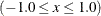, and they are typically continuous.

EXP

exponentiates variables (x is transformed to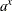). To specify the value of a, use the PARAMETER= t-option. By default, a is the mathematical constant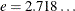. Variables specified with the EXP transform must be numeric, and they are typically continuous.

LOG

transforms variables to logarithms (x is transformed to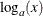). To specify the base of the logarithm, use the PARAMETER= t-option. The default is a natural logarithm with base. Variables specified with the LOG transform must be numeric and positive, and they are typically continuous.

LOGIT

finds a logit transformation on the variables. The logit of x is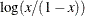. Unlike other transformations, LOGIT does not have a three-letter abbreviation. Variables specified with the LOGIT transform must be numeric and in the interval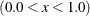, and they are typically continuous.

POWER
POW

raises variables to a specified power (x is transformed to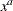). You must specify the power parameter a by specifying the PARAMETER= t-option following the variables.

power(variable / parameter=number)


You can use POWER for squaring variables (PARAMETER=2), reciprocal transformations (PARAMETER=–1), square roots (PARAMETER=0.5), and so on. Variables specified with the POWER transform must be numeric, and they are typically continuous.

RANK
RAN

transforms variables to ranks. Ranks are averaged within ties. The smallest input value is assigned the smallest rank. Variables specified with the RANK transform must be numeric.

##### Optimal Transformations

Optimal transformations are iteratively derived. Missing values for these types of variables can be optimally estimated (see the section Missing Values). See the sections OPSCORE, MONOTONE, UNTIE, and LINEAR Transformations and SPLINE and MSPLINE Transformations in Chapter 97: The TRANSREG Procedure, for more information about the optimal transformations.

The following list provides syntax and details for optimal transformations.

LINEAR
LIN

finds an optimal linear transformation of each variable. For variables with no missing values, the transformed variable is the same as the original variable. For variables with missing values, the transformed nonmissing values have a different scale and origin than the original values. Variables specified with the LINEAR transform must be numeric.

MONOTONE
MON

finds a monotonic transformation of each variable, with the restriction that ties are preserved. The Kruskal (1964) secondary least squares monotonic transformation is used. This transformation weakly preserves order and category membership (ties). Variables specified with the MONOTONE transform must be numeric, and they are typically discrete.

MSPLINE
MSP

finds a monotonically increasing B-spline transformation with monotonic coefficients (de Boor, 1978; de Leeuw, 1986) of each variable. You can specify the DEGREE=, KNOTS=, NKNOTS=, and EVENLY= t-options with MSPLINE. By default, PROC PRINQUAL uses a quadratic spline. Variables specified with the MSPLINE transform must be numeric, and they are typically continuous.

OPSCORE
OPS

finds an optimal scoring of each variable. The OPSCORE transformation assigns scores to each class (level) of the variable. The Fisher (1938) optimal scoring method is used. Variables specified with the OPSCORE transform can be either character or numeric; numeric variables should be discrete.

SPLINE
SPL

finds a B-spline transformation (de Boor, 1978) of each variable. By default, PROC PRINQUAL uses a cubic polynomial transformation. You can specify the DEGREE=, KNOTS=, NKNOTS=, and EVENLY t-options with SPLINE. Variables specified with the SPLINE transform must be numeric, and they are typically continuous.

UNTIE
UNT

finds a monotonic transformation of each variable without the restriction that ties are preserved. PROC PRINQUAL uses the Kruskal (1964) primary least squares monotonic transformation method. This transformation weakly preserves order but not category membership (it might untie some previously tied values). Variables specified with the UNTIE transform must be numeric, and they are typically discrete.

##### Other Transformations
IDENTITY
IDE

specifies variables that are not changed by the iterations. The IDENTITY transformation is used for variables when no transformation and no missing data estimation are desired. However, the REFLECT, ADDITIVE, TSTANDARD=Z, and TSTANDARD=CENTER options can linearly transform all variables, including IDENTITY variables, after the iterations. Observations with missing values in IDENTITY variables are excluded from the analysis, and no optimal scores are computed for missing values in IDENTITY variables. Variables specified with the IDENTITY transform must be numeric.

SSPLINE
SSP

finds an iterative smoothing spline transformation of each variable. The SSPLINE transformation does not generally minimize squared error. You can specify the smoothing parameter with either the SM= t-option or the PARAMETER= t-option. The default smoothing parameter is SM=0. Variables specified with the SSPLINE transform must be numeric, and they are typically continuous.

#### Transformation Options (t-options)

If you use a nonoptimal, optimal, or other transformation, you can use t-options, which specify additional details of the transformation. The t-options are specified within the parentheses that enclose variables and are listed after a slash. For example:

proc prinqual;
transform spline(X Y / nknots=3);
run;


The preceding statements find an optimal variable transformation (SPLINE) of the variables X and Y and use a t-option to specify the number of knots (NKNOTS=). The following is a more complex example:

proc prinqual;
transform spline(Y / nknots=3) spline(X1 X2 / nknots=6);
run;


These statements use the SPLINE transformation for all three variables and use t-options as well; the NKNOTS= option specifies the number of knots for the spline.

The following sections discuss the t-options available for nonoptimal, optimal, and other transformations.

Table 74.3 summarizes the t-options.

Table 74.3: Transformation Options

Option

Description

Nonoptimal Transformation

Uses original mean and variance

Parameter Specification

Specifies miscellaneous parameters

Specifies smoothing parameter

Spline

Specifies the degree of the spline

Spaces the knots evenly

Specifies the interior knots or break points

Creates n knots

Other t-options

Renames variables

Reflects the variable around the mean

Specifies transformation standardization

##### Nonoptimal Transformation t-options
ORIGINAL
ORI

matches the variable’s final mean and variance to the mean and variance of the original variable. By default, the mean and variance are based on the transformed values. The ORIGINAL t-option is available for all of the nonoptimal transformations.

##### Parameter t-options
PARAMETER=number
PAR=number

specifies the transformation parameter. The PARAMETER= t-option is available for the EXP, LOG, POWER, SMOOTH, and SSPLINE transformations. For EXP, the parameter is the value to be exponentiated; for LOG, the parameter is the base value; and for POWER, the parameter is the power. For SMOOTH and SSPLINE, the parameter is the raw smoothing parameter. (See the SM= option for an alternative way to specify the smoothing parameter.) The default for the PARAMETER= t-option for the LOG and EXP transformations is. The default parameter for SSPLINE is computed from SM=0. For the POWER transformation, you must specify the PARAMETER= t-option; there is no default.

SM=n

specifies a smoothing parameter in the range 0 to 100, just like PROC GPLOT uses. For example, SM=50 in PROC PRINQUAL is equivalent to I=SM50 on the SYMBOL statement with PROC GPLOT. You can specify the SM= t-option only with the SSPLINE transformation. The smoothness of the function increases as the value of the smoothing parameter increases. By default, SM=0.

##### Spline t-options

The following t-options are available with the SPLINE and MSPLINE optimal transformations.

DEGREE=n
DEG=n

specifies the degree of the B-spline transformation. The degree must be a nonnegative integer. The defaults are DEGREE=3 for SPLINE variables and DEGREE=2 for MSPLINE variables.

The polynomial degree should be a small integer, usually 0, 1, 2, or 3. Larger values are rarely useful. If you have any doubt as to what degree to specify, use the default.

EVENLY<=n>
EVE<=n>

is used with the NKNOTS= t-option to space the knots evenly. The differences between adjacent knots are constant. If you specify NKNOTS=k, k knots are created at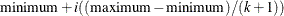for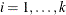. For example, if you specify

spline(X / knots=2 evenly)


and the variable X has a minimum of 4 and a maximum of 10, then the two interior knots are 6 and 8. Without the EVENLY t-option, the NKNOTS= t-option places knots at percentiles, so the knots are not evenly spaced.

By default for the SPLINE and MSPLINE transformations, the smaller exterior knots are all the same and just a little smaller than the minimum. Similarly, by default, the larger exterior knots are all the same and just a little larger than the maximum. However, if you specify EVENLY=n, then the n exterior knots are evenly spaced as well. The number of exterior knots must be greater than or equal to the degree. You can specify values larger than the degree when you want to interpolate slightly beyond the range or your data. The exterior knots must be less than the minimum or greater than the maximum, and hence the knots across all sets are not precisely equally spaced. For example, with data ranging from 0 to 10, and with EVENLY=3 and NKNOTS=4, the first exterior knots are –4.000000000001, –2.000000000001, and –0.000000000001, the interior knots are 2, 4, 6, and 8, and the second exterior knots are 10.000000000001, 12.000000000001, and 14.000000000001.

KNOTS=number-list | n TO m BY p
KNO=number-list | n TO m BY p

specifies the interior knots or break points. By default, there are no knots. The first time you specify a value in the knot list, it indicates a discontinuity in the nth (from DEGREE=n) derivative of the transformation function at the value of the knot. The second mention of a value indicates a discontinuity in the (n – 1) derivative of the transformation function at the value of the knot. Knots can be repeated any number of times to decrease the smoothness at the break points, but the values in the knot list can never decrease.

You cannot use the KNOTS= t-option with the NKNOTS= t-option. You should keep the number of knots small. (See the section Specifying the Number of Knots in Chapter 97: The TRANSREG Procedure.)

NKNOTS=n
NKN=n

creates n knots, the first at the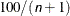percentile, the second at the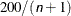percentile, and so on. Knots are always placed at data values; there is no interpolation. For example, if NKNOTS=3, knots are placed at the 25th percentile, the median, and the 75th percentile. By default, NKNOTS=0. The NKNOTS= t-option must be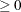.

You cannot use the NKNOTS= t-option with the KNOTS= t-option. You should keep the number of knots small. (See the section Specifying the Number of Knots in Chapter 97: The TRANSREG Procedure.)

##### Other t-options

The following t-options are available for all transformations.

NAME=(variable-list)
NAM=(variable-list)

renames variables as they are used in the TRANSFORM statement. This option allows a variable to be used more than once. For example, if the variable X is a character variable, then the following step stores both the original character variable X and a numeric variable XC that contains category numbers in the output data set.

proc prinqual data=A n=1 out=B;
transform linear(Y) opscore(X / name=(XC));
id X;
run;

REFLECT
REF

reflects the transformation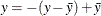after the iterations are completed and before the final standardization and results calculations.

TSTANDARD=CENTER | NOMISS | ORIGINAL | Z
TST=CEN | NOM | ORI | Z

specifies the standardization of the transformed variables in the OUT= data set. By default, TSTANDARD=ORIGINAL. When the TSTANDARD= option is specified in the PROC PRINQUAL statement, it specifies the default standardization for all variables. When you specify TSTANDARD= as a t-option, it overrides the default standardization only for selected variables.